# Simple Logic Gates 2.1.11 2.1.13

2.1.11 2.1.13

## Challenge 1  Logic Gates

Translate the the following logical expression into both a Truth Table and a Logic Diagram

(a or b) and ( a nand b)

Q1 Identify a single logic gate that is the equivalent

Q2 Outline how truth tables can be used to test if any 2 logic circuits are equivalent

Challenge 2  Logic Programming operators

You can copy the code below and play about with different logic operators. Fix the program so it works based on the propositions.

Q3 Use you imagination to define 3 propositions that need to be true for an action to take. Write the equivalent  PHP code . Post your source code to you google site

POST your ph code to your Google Web Site page on same page (  Option 2 Logic gates)2.1.11 Define the Boolean operators: AND, OR, NOT, NAND, NOR and XOR.

Spend time changing the inputs to each gate, stop when you feel confident in what will happen based on the inputs for each gate ( practice  link below ) ( this may not work if not proceed to next step )

## Using XOR and AND Gates to add  2 binary digits

The half adder produces a sum (S) and a carry value (C) which are both binary digits.

The block diagram of a half adder is shown below in fig.1.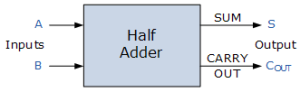Fig.1

# ​

Half Adder Truth Table with Carry-Out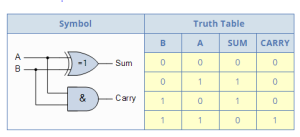In boolean Logic

A ⊕  B = Sum

A * B = Carry

Why is it called a Half Adder ?

2.1.12 Construct truth tables using the above operators and Equivalence.

### How to Tell if Two Different Logical Statements are Equivalent

To tell if two logical statements are equivalent or not, we need to create a truth table for each statement, and compare the truth values of the statements in each case. If both statements have truth tables with exactly the same truth values in the final column, then the two statements are logically equivalent, and one statement can be replaced with the other in a logical argument without changing the meaning.

Let's look at some examples:

#### Example 1

Is ~(pq) equivalent to ~p∧~q?

Because we know that in arithmetic 2(x+3)=2x+6, we may start to wonder if we can distribute the negation sign over a set of parentheses in logical statements. In other words, is ~(pq) equivalent to ~p∧~q?

The only way for us to find out is to make a truth table for ~(pq) and a truth table for ~p∧~q and then compare the truth values in each table:

 p q p∧q ~(p∧q) T T T F T F F T F T F T F F F T
 p q ~p ~q ~p∧~q T T F F F T F F T F F T T F F F F T T T

In order for this comparison to work, we have to compare the same row in each truth table; for example, we must compare the row where p=T and q=T in the truth table for ~(p∧q) with the row where p=T and q=T in the truth table for ~p∧~q. We are interested in whether or not the two different statements have the same truth value in exactly the same conditions. So if we were to compare the row where p=T and q=T in the truth table for ~(p∧q) with the row where p=T and q=F in the truth table for ~p∧~q, we would be looking at two different sets of conditions, and so comparing the truth values for each statement in this case would lead to an error.

In the two truth tables I've created above, you can see that I've listed all the truth values of p and q in the same order. This is so that I can compare the values in the final column in the two truth tables without worrying about whether or not I am matching up the right rows - because the rows are already in the same order, I can just compare the final column of one table with the final column of the other like this:

 p q ~(p∧q) ~p∧~q T T F F T F T F F T T F F F T T

In this case, the truth values for ~(p∧q) and ~p∧~q are not exactly the same, so we can conclude that the two statements are not equivalent!

So, this answers our question; if we have ~(p∧q), we cannot simply replace it with ~p∧~q. If we replace ~(p∧q) with ~p∧~q, we will actually be changing the meaning of our statement.

But is there some other way to get rid of the parentheses? In other words, is there some other statement which is equivalent to ~(p∧q) which does not contain any parentheses? Let's try another possibility:

#### Example 2:

Is ~(pq) equivalent to ~p∨~q?

To test this statement, we must make a truth table for ~(pq) and a truth table for ~p∨~q and then compare the truth values in each table:

 p q p∧q ~(p∧q) T T T F T F F T F T F T F F F T
 p q ~p ~q ~p∨~q T T F F F T F F T T F T T F T F F T T T

In the two truth tables I've created above, you can see that I've listed all the truth values of p and q in the same order. This is so that I can compare the values in the final column in the two truth tables without worrying about whether or not I am matching up the right rows - because the rows are already in the same order, I can just compare the final column of one table with the final column of the other like this:

 p q ~(p∧q) ~p∨~q T T F F T F T T F T T T F F T T

In this case, the truth values for ~(p∧q) and ~p∨~q are exactly the same, so we can conclude that the two statements are equivalent:

~(p∧q)≡~p∨~q

So, if we ever encounter ~(p∧q), we can replace it with ~p∨~q without changing the logical meaning of the statement!

## Logic gates: circuits that compare

A logic gate might sound horribly complex, but it's simply an electric circuit with two inputs and an output. It receives two incoming electric currents, compares them, and sends on a new, outgoing electric current depending on what it finds. A logic gate is a bit like a doorman or bouncer who is allowed to let people into a nightclub only if they pass certain tests. There are quite a few different types of logic gate, the most common of which are called AND, OR, NOT, XOR (Exclusive Or), NAND (NOT AND), and NOR (NOT OR). Let's look at the three simpler ones, AND, OR, and NOT:

### AND

Suppose you go to a nightclub where the doorman's job is to enforce a simple rule: "Everyone in your group must wear a tie to come in". You go along with a friend one night. If you're both wearing ties, you'll get in. If only one of you is wearing a tie, or if neither of you is, neither of you will get in. An AND logic gate works the same way with two electrical inputs. If both inputs are switched on (that is, carry a number 1), the output will be 1 as well. Otherwise the output will be 0. In electronics, we can represent an AND gate with this little symbol. Three ways in which the gate can work are shown below.### OR

You're not wearing a tie, so you go to another club further down the street. Here, the person on the door is enforcing a different rule: "A group of people can come in if any one of them is a member". If either you or your friend is a member, or if you both are members, you can both come in. If neither of you is a member, you're both left out in the cold. An OR logic gate works this way with two electrical inputs. If either input is switched on (that is, carries a number 1), the output will be 1 as well. Otherwise the output will be 0. In electronics, we represent an OR gate with a different symbol. Three ways in which it can work are shown beneath: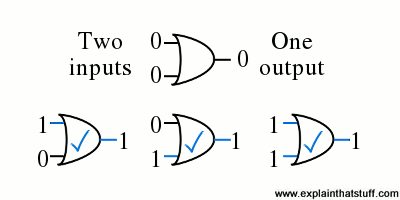### NOT

So far, you've failed to get into either of the clubs. But there's one last hope: you know a friend is having a party a few streets away. The only trouble is, there's a really argumentative and contrary person on the door. He talks to each person in turn as they approach him. If you're nice and polite, he shouts abuse at you and turns you away. But if you're rude to him, he likes that for some reason and lets you in. In other words, he does exactly the opposite of what you'd expect! In electronics, there's a logic gate that works in the same, contrary way and it's called a NOT gate or inverter. Unlike AND and OR gates, it has only one input and one output. The output is exactly the opposite of the input, so if the input is a 0, the output is a 1 and vice versa. Here's how we represent a NOT in electronics. Two ways it can work are shown beneath.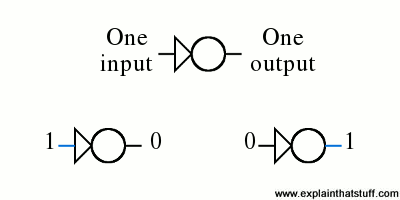The other three common logic gates are variations on these three. XOR (Exclusive OR) is like an OR, but it switches off if both the inputs are switched on. NAND is just like AND, only the end result is swapped over (so where AND produces an output of 1, NAND produces an output of 0). NOR is like OR with the end result swapped over in the same way.

This logical way of comparing numbers to make decisions that produce either a yes or no, 1 or 0, true or false is called Boolean algebra after its discoverer, English mathematician George Boole (1815–1864), who set out the idea in an 1854 book titled An Investigation of the Laws of Thought, on Which Are Founded the Mathematical Theories of Logic and Probabilities. His objective was to show how complex human reasoning could be represented in a logical, mathematical form.

## Controlling a calculator display with logic gates

Calculators can do all the things they need to do using different combinations of logic gates. It's logic gates that control how the display works in a calculator and more logic gates that figure out the results of calculations.

For example, each segment of a calculator's display is switched on and off by a series of logic gates that are connected together. Consider just the bottom lower right segment (shown in the picture, right, in blue). We need to turn this segment on if we're showing the numbers 0 (binary 00), 1 (01), 3 (11), 4 (100), 5 (101), 6 (110), 7 (111), 8 (1000), and 9 (1001)—but not if we're showing the number 2 (10). We can make the segment switch on and off correctly for the numbers 1–10 by rigging up three OR gates and one NOT gate like this.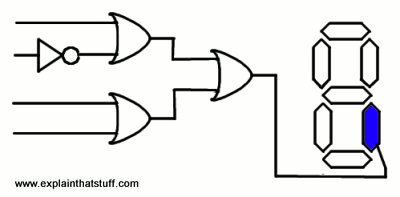If we feed the patterns of binary numbers into the four inputs on the left, the segment will turn on and off correctly for each one. For example, feed in the number 7 as the four inputs 0111 and the gates will trigger like this, correctly switching on the segment:The other six segments of each digit are controlled by other combinations of logic gates.

Challenge A Logic Gates

## Visual Reprenstation of Logic gates